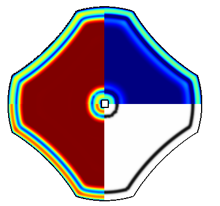# 论文和技术资料

## Topology Optimization of Thermoviscous Acoustics in Tubes and Slits with Hearing Aid Applications

R. Christensen ,
 GN ReSound A/S, Ballerup, Copenhagen, Denmark

When acoustic applications include small geometries, i.e. mm/submillimeter range, so-called thermoviscous effects can greatly affect the pressure response. This paper deals with acoustic topology optimization of such miniature structures with thermoviscous effects taken into account.

The most general method for calculating acoustics with thermoviscous effects involves solving the Full Linearized Navier-Stokes (FLNS) equation set, where the velocity vector field, the scalar pressure field and the scalar temperature (variation) field are coupled. The equation set in its general form does not easily lend itself to topology optimization, and no attempts at this are found in the available literature. There are, however, approximate methods which are accurate for typical applications and frequency ranges, such as acoustics for hearing aid audio. One method  in particular was found useful, since it involves solving a set of Helmholtz equations, for which both the velocity field and the thermal field have been decoupled from the acoustic pressure: The velocity and the temperature are both described via the equation

Ψϕ+kϕ^(-2) ∆Ψ_ϕ=1 (Eq. 1)

where kϕ is a wavenumber, and Ψϕ is a variable related to either temperature (ϕ=h) or velocity (ϕ=v). Both the temperature and the velocity field are combined wave and diffusion processes, and this nature is described via the complex and frequency-dependent wavenumber. The build-in damping nature of the problem could help also stabilize the optimization process. For straight tubes, the Ψ_ϕ-field is fairly simple, and so it should be possible to set up an optimization routine for such geometries. The averaged (over the cross-section of the tube) fields can be directly linked to transmission line parameters, which in turn completely describe the acoustic behavior.

Isentropic (i.e. standard) acoustic topology optimization involves finding an optimum, and preferably binary, distribution of air and “solid-like” material, where a design (parameter) determines the density and bulk modulus of the material, within given constraints. The sound field is typically quite complex, and finding an optimum solution can be a challenge.

With the proposed thermoviscous model a topology optimization scheme is set up for straight tubes, where an interpolation between air and “solid” is obtained, using interpolations of both the source term in (Eq.1) as well as the absorption coefficient. An example is shown in the attached figure, where a circular cross-section is optimized to have the highest “loss-to-acoustic mass” ratio possible within given constraints. Filtering has yet to be applied to the optimization routine, so mesh sensitivity is seen.

The implementation in COMSOL Multiphysics® requires PDE physics interfaces combined with the Optimization and Sensitivity physics interfaces.

The proposed method is the first to offer a practical way of doing acoustic topology optimization with viscothermal losses accurately included. Typical applications of relevance are hearing aids, mobile phones/tablets and other communication equipment containing narrow regions.

 R. Kampinga, “Viscothermal Acoustics Using Finite Elements: Analysis Tools for Engineers”, PhD thesis, University of Twente, 2010